# A9 9420

Performance
46.33k points

Description
The A9 9420 is a cpu manufactured by AMD that was released on April 2017. This model has 2 Stoney Ridge cores , runs at 3000 MHz as base frequency and has a a thermal design power of 15 W.

Specs
Official name
AMD A9-9420 RADEON R5, 5 COMPUTE CORES 2C+3G
CPUID
670f00
Core
Stoney Ridge
Architecture
Base frecuency
3 GHz
Boost frecuency
3.6 GHz
Socket
Micro-BGA
2/2
TDP
15 W
Cache L1 (d+i)
96+2x32 kB
Cache L2
1024 kB
Cache L3
0 kB
Release date
April 2017
33.09k points
46.33k points

Non-optimized benchmark
The benchmark in Mode 0 (FPU) measures cpu performance with non-optimized software. It uses the basic µinstructions from the i386 architecture with the i387 floating point unit. This mode is compatible with all CPUs so it's practical to compare very different CPUs
Test#1 (Integers)
2.56k
3.88k (x1.5)
Test#2 (FP)
9.97k
9.26k (x0.9)
Test#3 (Generic, ZIP)
2.96k
3.9k (x1.3)
Test#1 (Memory)
2.12k
1.74k (x0.8)
TOTAL
17.61k
18.79k (x1.1)

SSE3 optimized benchmark
The benchmark in mode I (SSE) is optimized for the use of SIMD instructions with 128 bits register and the SSE set up to version 3. Nearly every modern CPU has support for this mode.
Test#1 (Integers)
8.41k
11.25k (x1.3)
Test#2 (FP)
11.48k
13.69k (x1.2)
Test#3 (Generic, ZIP)
3.29k
2.09k (x0.6)
Test#1 (Memory)
2.24k
1.87k (x0.8)
TOTAL
25.41k
28.89k (x1.1)

AVX optimized benchmark
The benchmark in mode II (AVX) is optimized to used 256 bits registers beside the first version of the Advanced Vector Extensions (AVX). The first AVX compatible CPU was released in 2011.
Test#1 (Integers)
7.55k
9.09k (x1.2)
Test#2 (FP)
13.9k
12.25k (x0.9)
Test#3 (Generic, ZIP)
3.11k
3k (x1.0)
Test#1 (Memory)
2.18k
1.81k (x0.8)
TOTAL
26.74k
26.15k (x1.0)

AVX2 optimized benchmark
The benchmark in mode III (AVX2), like AVX1, is optimized to used 256 bits registers beside the second version of the Advanced Vector Extensions (AVX). The first AVX2 compatible CPU was released in 2013.
Test#1 (Integers)
11.74k
18.57k (x1.6)
Test#2 (FP)
16.05k
20.11k (x1.3)
Test#3 (Generic, ZIP)
3.26k
5.27k (x1.6)
Test#1 (Memory)
2.05k
2.38k (x1.2)
TOTAL
33.09k
46.33k (x1.4)

Computers where this CPU was tested
Model
Release date

RAM models tested along with this CPU
Model
Type
Frequency
Size
DDR4
1866 MHz
8 GB

Monothread performance graphics gives the performance vs time. They are useful to measure the time it takes to the CPU to reach the maximum performance.

Usually, CPU's performance will be steady during these tests but if it has a slow frequency strategy, the first samples will show a lower score.

Test#1 (Integers) [% vs time]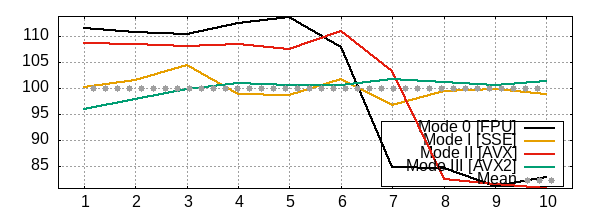Test#2 (FP) [% vs time]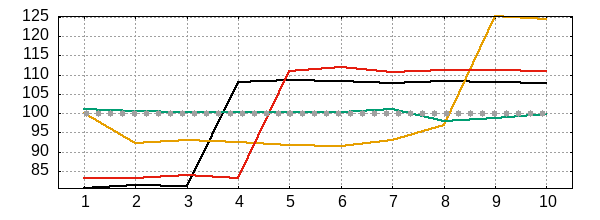Test#3 (Generic, ZIP) [% vs time]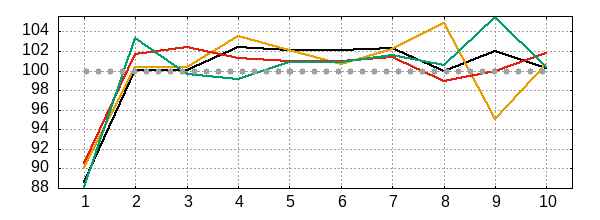Test#1 (Memory) [% vs time]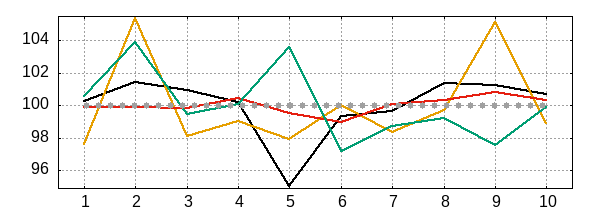Multithread graphs measure the performance against a heavy load during certain time.

If CPU's TDP doesn't limit the frequency and the machine is properly cooled, performance should remain steady vs time. Otherwise, the performance score will oscillate or decrease over time.

Test#1 (Integers) [% vs time]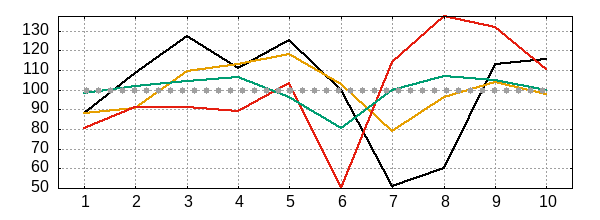Test#2 (FP) [% vs time]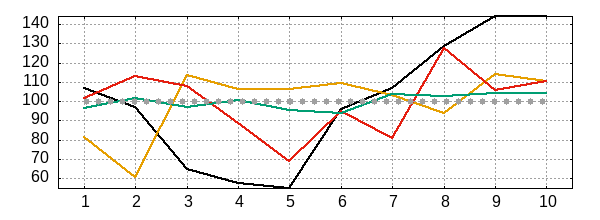Test#3 (Generic, ZIP) [% vs time]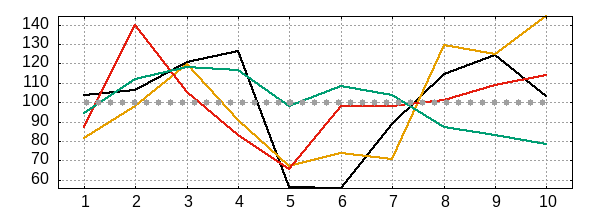Test#1 (Memory) [% vs time]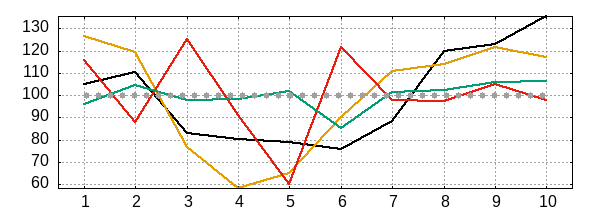Similar CPUs to A9 9420
A9 9420 (This model)
Mode FPU monothread  [11.93k points -32.28%]
Mode FPU multithread  [25.84k points +37.51%]
Mode AVX2 monothread  [21.57k points -34.82%]
Mode AVX2 multithread  [46.09k points -0.52%]
Mode AVX2 monothread  [31.31k points -5.39%]
Mode AVX2 multithread  [41.73k points -9.94%]
Mode FPU monothread  [16.35k points -7.16%]
Mode FPU multithread  [31.86k points +69.58%]
Mode AVX2 monothread  [27.62k points -16.52%]
Mode AVX2 multithread  [58.51k points +26.27%]
Mode FPU monothread  [18.21k points +3.38%]
Mode FPU multithread  [39.24k points +108.83%]
Mode AVX2 monothread  [35.13k points +6.17%]
Mode AVX2 multithread  [79.15k points +70.81%]
Mode FPU monothread  [18.86k points +7.07%]
Mode FPU multithread  [37.05k points +97.18%]
Mode AVX2 monothread  [33.38k points +0.87%]
Mode AVX2 multithread  [79.6k points +71.80%]
Mode AVX monothread  [14.65k points -55.73%]
Mode AVX multithread  [30.46k points -34.27%]
Mode FPU monothread  [14.79k points -15.99%]
Mode FPU multithread  [123.78k points +558.80%]
Mode AVX2 monothread  [29.39k points -11.17%]
Mode AVX2 multithread  [237.83k points +413.29%]
Mode AVX2 monothread  [29.34k points -11.32%]
Mode AVX2 multithread  [52.42k points +13.13%]
Mode FPU monothread  [15.62k points -11.31%]
Mode FPU multithread  [31.35k points +66.86%]
Mode AVX monothread  [20.1k points -39.25%]
Mode AVX multithread  [38.68k points -16.52%]
Mode FPU monothread  [16.27k points -7.63%]
Mode FPU multithread  [23.25k points +23.76%]
Mode SSE monothread  [16.91k points -48.90%]
Mode SSE multithread  [26.88k points -41.98%]

FPU mode comparison

Model
Score
2.63k [2.45%]
2.63k [2.45%]
AMD A9 9420
2.56k
This model

Model
Score
AMD A9 9420
3.88k
This model
3.83k [-1.48%]

Model
Score
10.58k [6.15%]
AMD A9 9420
9.97k
This model
9.77k [-2.02%]
9.64k [-3.30%]

Model
Score
AMD A9 9420
9.26k
This model
9.13k [-1.37%]
8.55k [-7.72%]
7.69k [-17.00%]

Model
Score
AMD A9 9420
2.96k
This model
2.82k [-4.58%]
2.75k [-6.87%]

Model
Score
2.42k [14.09%]
2.41k [13.42%]
AMD A9 9420
2.12k
This model
2.01k [-5.11%]
1.88k [-11.28%]

Model
Score
2.01k [15.58%]
AMD A9 9420
1.74k
This model
1.52k [-12.81%]
1.45k [-16.39%]

SSE mode comparison

Model
Score
8.77k [4.26%]
AMD A9 9420
8.41k
This model
8.39k [-0.25%]
8.34k [-0.86%]
8.29k [-1.42%]

Model
Score
12.27k [9.10%]
11.95k [6.26%]
11.78k [4.69%]
AMD A9 9420
11.25k
This model
11.19k [-0.54%]
10.06k [-10.60%]
10.02k [-10.97%]

Model
Score
11.82k [3.02%]
11.82k [2.96%]
11.68k [1.78%]
11.67k [1.68%]
AMD A9 9420
11.48k
This model
11.35k [-1.06%]
10.55k [-8.03%]

Model
Score
13.83k [1.05%]
13.75k [0.48%]
AMD A9 9420
13.69k
This model
13.48k [-1.49%]
13.17k [-3.79%]

Model
Score
3.47k [5.61%]
3.35k [1.90%]
AMD A9 9420
3.29k
This model
3.28k [-0.31%]
3.26k [-0.86%]

Model
Score
2.85k [36.61%]
2.46k [17.98%]
2.42k [15.99%]
AMD A9 9420
2.09k
This model
1.92k [-7.94%]

Model
Score
2.33k [4.28%]
AMD A9 9420
2.24k
This model
2.18k [-2.66%]
2.13k [-4.66%]
2.05k [-8.17%]
2.03k [-9.15%]

Model
Score
2.09k [11.70%]
AMD A9 9420
1.87k
This model
1.86k [-0.36%]
1.73k [-7.30%]

AVX mode comparison

Model
Score
7.65k [1.37%]
AMD A9 9420
7.55k
This model
7.34k [-2.72%]
7.33k [-2.86%]

Model
Score
11.92k [31.14%]
11.67k [28.33%]
10.88k [19.68%]
9.55k [5.04%]
AMD A9 9420
9.09k
This model
9.01k [-0.92%]
8.43k [-7.30%]
7.72k [-15.06%]
3.3k [-63.73%]

Model
Score
16.98k [22.15%]
15.33k [10.25%]
15.18k [9.19%]
AMD A9 9420
13.9k
This model
13.49k [-2.96%]
12.85k [-7.58%]
12.72k [-8.50%]
12.55k [-9.78%]

Model
Score
13.9k [13.46%]
13.51k [10.25%]
12.8k [4.47%]
AMD A9 9420
12.25k
This model
12.17k [-0.70%]
12.17k [-0.70%]
10.74k [-12.33%]
10.42k [-14.92%]
10.01k [-18.28%]

Model
Score
AMD A9 9420
3.11k
This model
3.1k [-0.42%]
3.05k [-1.93%]
2.97k [-4.60%]

Model
Score
4.64k [54.33%]
3.32k [10.45%]
AMD A9 9420
3k
This model
1.76k [-41.49%]

Model
Score
2.39k [9.66%]
2.39k [9.65%]
AMD A9 9420
2.18k
This model
2.05k [-5.88%]

Model
Score
2.16k [19.45%]
2.15k [18.84%]
AMD A9 9420
1.81k
This model
1.63k [-10.01%]
1.59k [-11.91%]
1.45k [-19.93%]
1.26k [-30.21%]
0.87k [-51.83%]

AVX2 mode comparison

Model
Score
12.89k [9.82%]
12.77k [8.77%]
12.44k [6.02%]
12.39k [5.61%]
AMD A9 9420
11.74k
This model
11.73k [-0.08%]
11.66k [-0.66%]
11.35k [-3.29%]
11.27k [-3.98%]
11.23k [-4.35%]

Model
Score
24.16k [30.10%]
22.14k [19.19%]
21.84k [17.60%]
21.75k [17.07%]
20.97k [12.91%]
AMD A9 9420
18.57k
This model
17.13k [-7.77%]
16.58k [-10.76%]
15.75k [-15.20%]
12.83k [-30.95%]
11.42k [-38.53%]

Model
Score
16.75k [4.37%]
16.52k [2.93%]
16.18k [0.81%]
AMD A9 9420
16.05k
This model
15.96k [-0.57%]
15.61k [-2.74%]
15.24k [-5.01%]
14.96k [-6.79%]

Model
Score
23.69k [17.82%]
22.25k [10.63%]
21.59k [7.38%]
20.51k [1.99%]
AMD A9 9420
20.11k
This model
19.15k [-4.75%]
17.92k [-10.90%]
17.84k [-11.29%]
15.86k [-21.12%]
14.44k [-28.20%]

Model
Score
3.33k [2.19%]
AMD A9 9420
3.26k
This model
3.17k [-2.52%]
3.15k [-3.41%]
3.11k [-4.51%]
3.08k [-5.54%]

Model
Score
6.74k [27.70%]
5.94k [12.66%]
5.87k [11.37%]
AMD A9 9420
5.27k
This model
5.09k [-3.53%]
4.97k [-5.83%]
3.62k [-31.44%]
3.6k [-31.67%]

Model
Score
2.24k [9.24%]
2.06k [0.39%]
AMD A9 9420
2.05k
This model
1.79k [-12.68%]
1.7k [-17.01%]
1.3k [-36.79%]